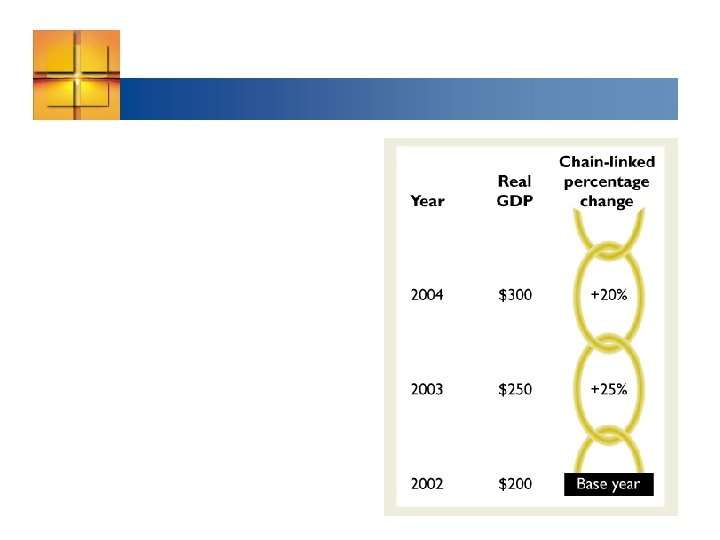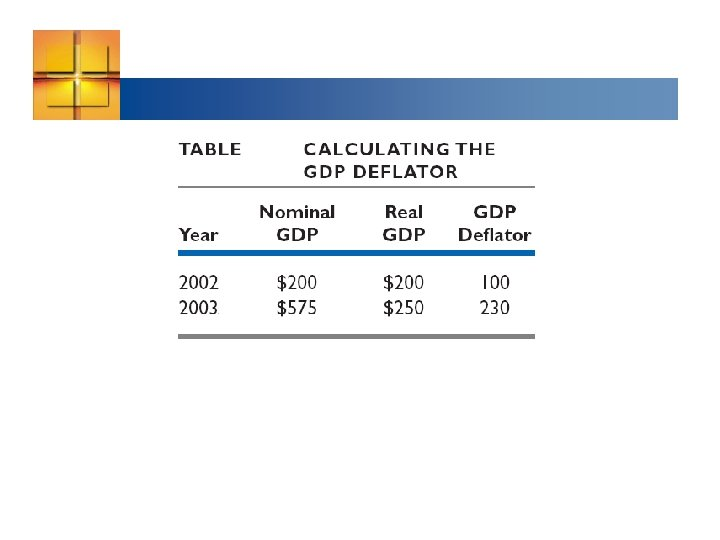Скачать презентацию Figure 20 1 shows the circular flow of

• Количество слайдов: 40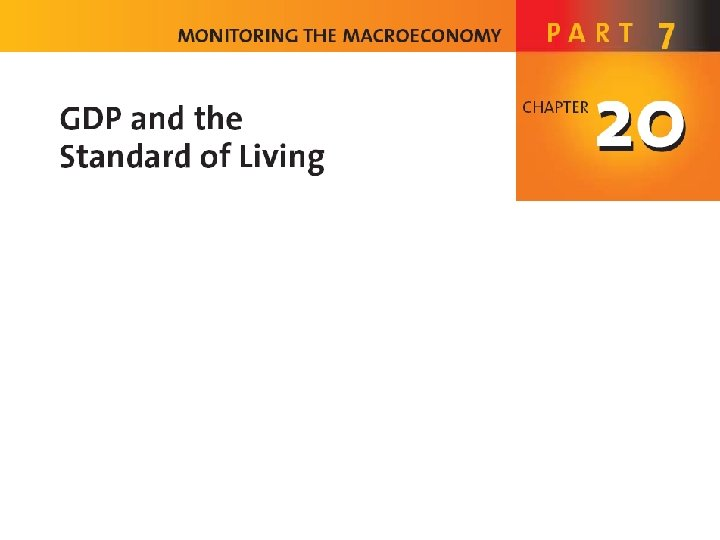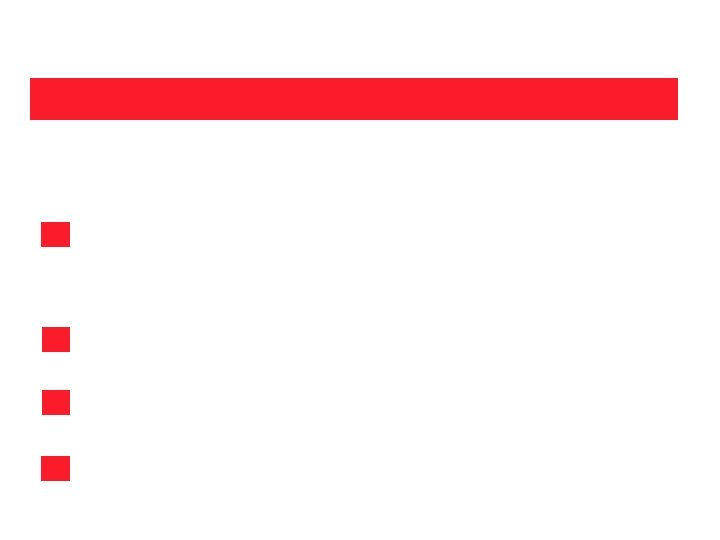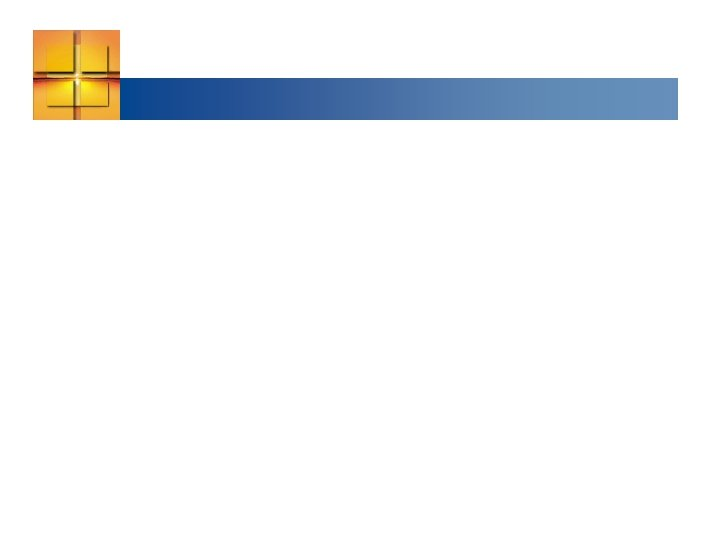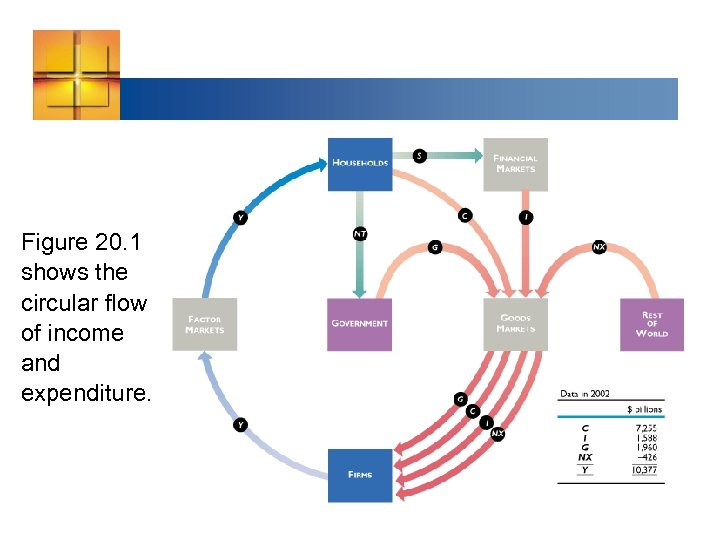Figure 20. 1 shows the circular flow of income and expenditure.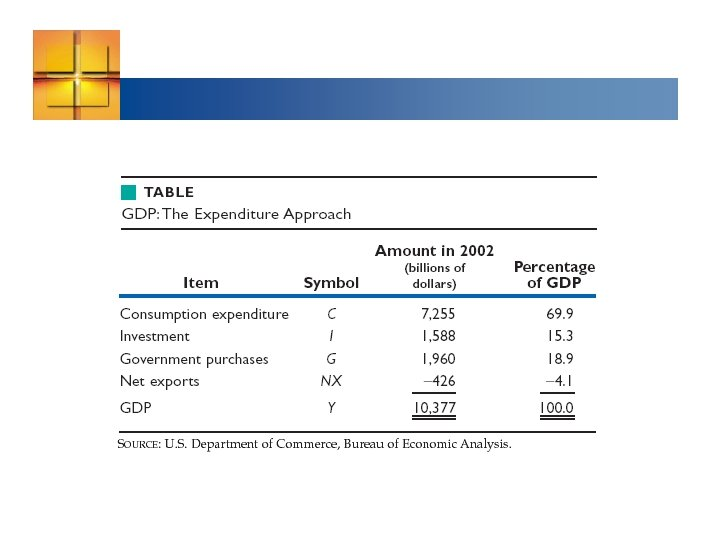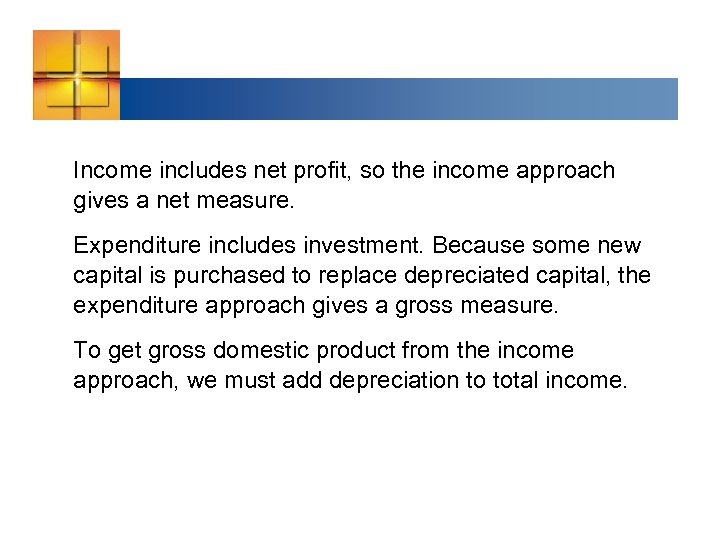Income includes net profit, so the income approach gives a net measure. Expenditure includes investment. Because some new capital is purchased to replace depreciated capital, the expenditure approach gives a gross measure. To get gross domestic product from the income approach, we must add depreciation to total income.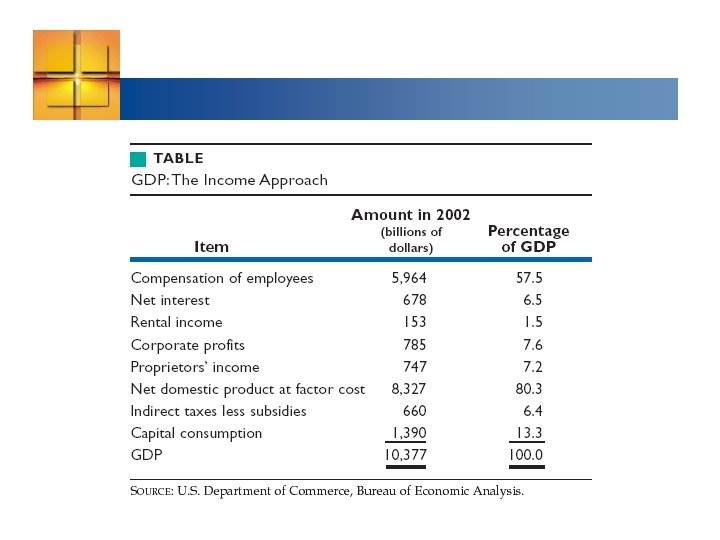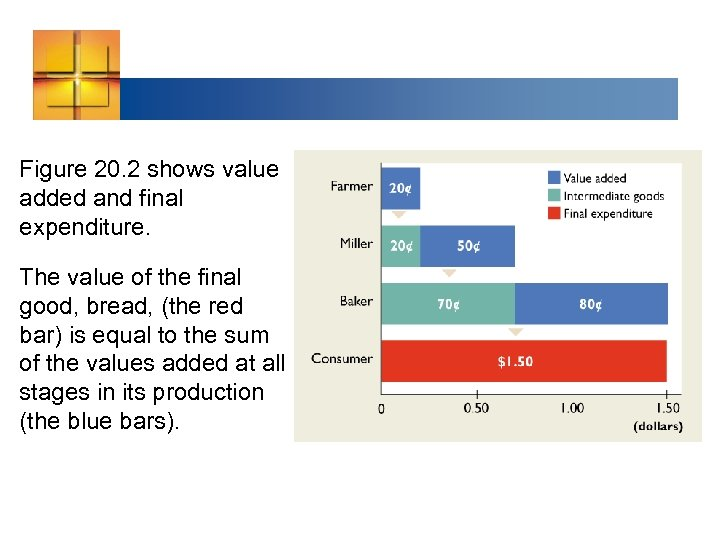Figure 20. 2 shows value added and final expenditure. The value of the final good, bread, (the red bar) is equal to the sum of the values added at all stages in its production (the blue bars).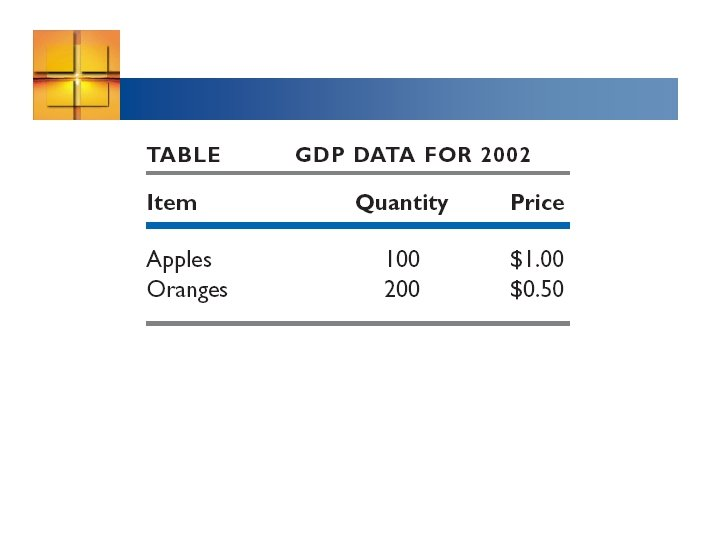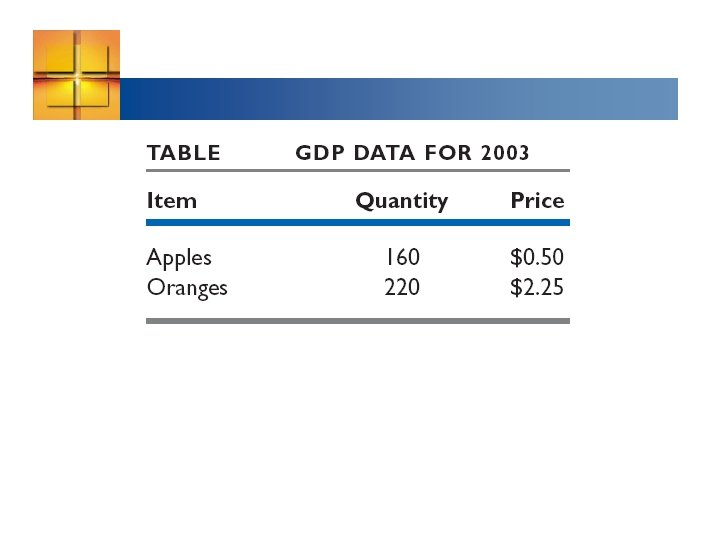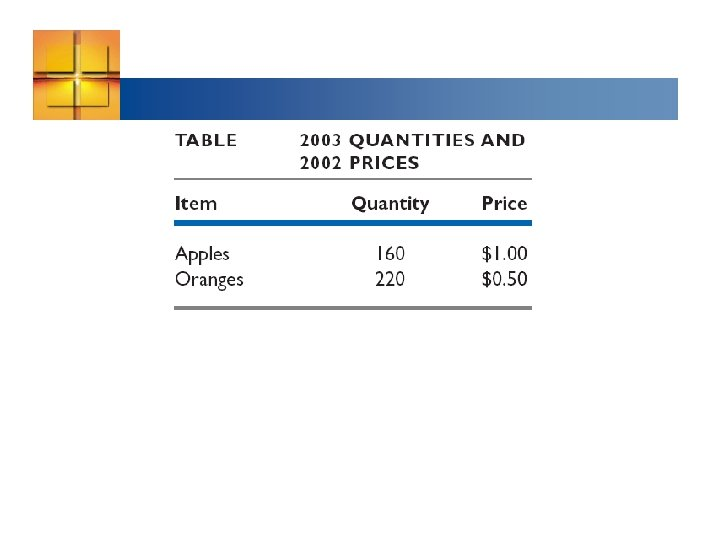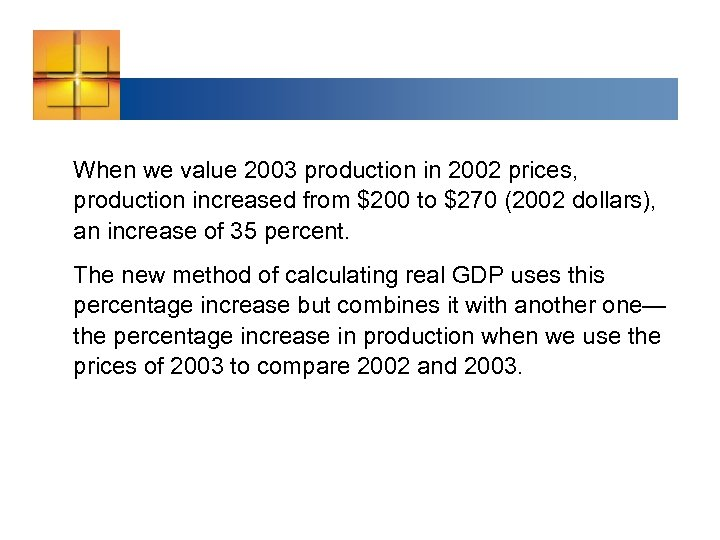When we value 2003 production in 2002 prices, production increased from \$200 to \$270 (2002 dollars), an increase of 35 percent. The new method of calculating real GDP uses this percentage increase but combines it with another one— the percentage increase in production when we use the prices of 2003 to compare 2002 and 2003.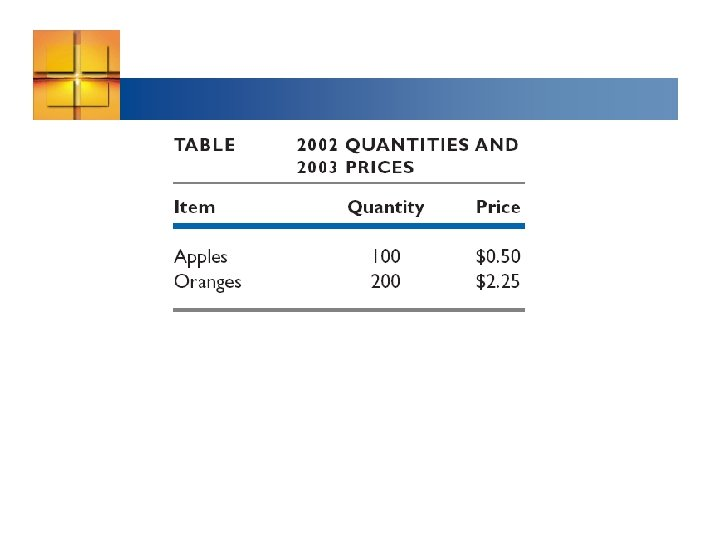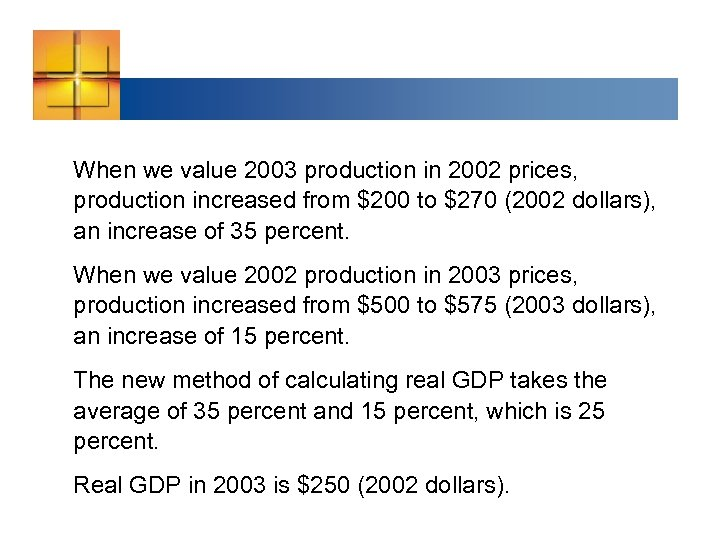When we value 2003 production in 2002 prices, production increased from \$200 to \$270 (2002 dollars), an increase of 35 percent. When we value 2002 production in 2003 prices, production increased from \$500 to \$575 (2003 dollars), an increase of 15 percent. The new method of calculating real GDP takes the average of 35 percent and 15 percent, which is 25 percent. Real GDP in 2003 is \$250 (2002 dollars).